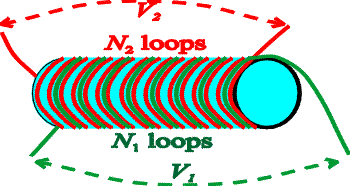Transformers

Transformers are used to change the voltage of an AC voltage source. This can be done by winding two sets of coils around the same core as shown below.Every loop of wire has the same flux through it, and hence the same rate of change in flux, and hence produces the same voltage. The voltage across each coil is therefore proportional to the number of turns in it, so the voltages are related by

V2 = (N2 / N1) V1

If the green loop is hooked to a power supply with voltage V1, the red wires will serve as a power supply with an increased voltage V2 if there are more red than green turns around the core.

No power is gained or lost in the transformer (we neglect the resistance of the wires), so the power going out of the transformer equals the power going in. Hence V1 I1 = V2 I2 . If the voltage increases, the current decreases; in general

I2 = (N1 / N2) I1

Note that transformers depend on changes in flux, so they work only for AC, not DC. The ease in changing voltages with transformers is the reason that AC is used for the distribution of electric power.

Examples       Induction index        Lecture index